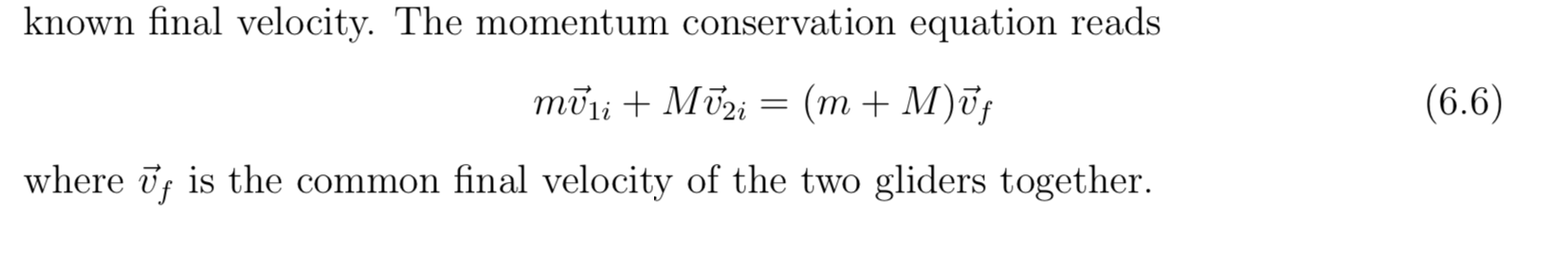# known final velocity. The momentum conservation equation reads(m + M)ūj(6.6)moliMiwhere f is the common final velocity of the two gliders together

Question

Compute the ratios of the intial velocities values below when final velocity is near zero with error of velocity ± 0.01m/s and weight 0.1g.

Mass1: 195.2g ± 0.1g

Mass2: 182.8 g ± 0.1g

 M1 Intial Velocity 0.32m/s Final -0.4m/s M2 Intial Velocity 0.43m/s Final Velocty -0.4m/shelp_outlineImage Transcriptioncloseknown final velocity. The momentum conservation equation reads (m + M)ūj (6.6) moliMi where f is the common final velocity of the two gliders together fullscreen
check_circleExpert Solution
Step 1

According to the conservation of momentum equation,

Substitute the value...

### Want to see the full answer?

See Solution

#### Want to see this answer and more?

Solutions are written by subject experts who are available 24/7. Questions are typically answered within 1 hour*

See Solution
*Response times may vary by subject and question
Tagged in

### Physics